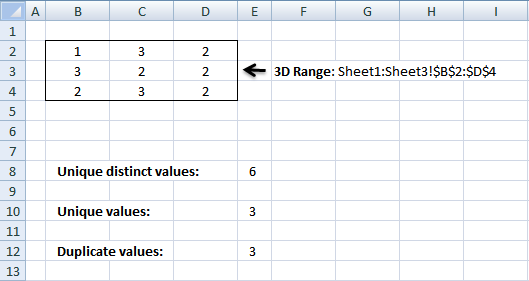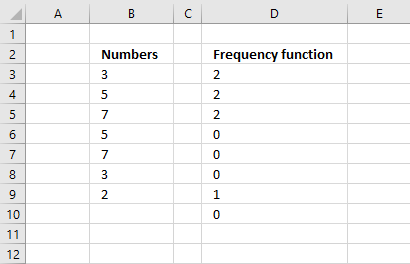Author: Oscar Cronquist Article last updated on December 18, 2018The image above demonstrates three different formulas in column E that counts

• unique numbers
• unique distinct numbers
• duplicate numbers

from multiple sheets in the same workbook.

### Count unique distinct numbers

The following formula counts unique distinct numbers in multiple sheets (3D range):

Formula in E8:

=SUMPRODUCT(--(FREQUENCY(Sheet1:Sheet3!\$B\$2:\$D\$4, Sheet1:Sheet3!\$B\$2:\$D\$4)<>0))

To enter an array formula, type the formula in a cell then press and hold CTRL + SHIFT simultaneously, now press Enter once. Release all keys.

The formula bar now shows the formula with a beginning and ending curly bracket telling you that you entered the formula successfully. Don't enter the curly brackets yourself.

### Explaining formula in cell E8

#### Step 1 - Count numbers

The FREQUENCY function calculates the number of times a number exists in a cell range, it also has the ability to count numbers across multiple worksheets.The FREQUENCY function returns the count for the corresponding number only once. Example, 3 exists twice in column B above so the function returns 2 on the same row, however, the next time 3 appears in the list the function returns 0 (zero), see row 8. We can use that to count numbers.

FREQUENCY(Sheet1:Sheet3!\$B\$2:\$D\$4, Sheet1:Sheet3!\$B\$2:\$D\$4)

returns

{1; 9; 7; 0; 0; 0; 0; 0; 0; 0; 8; 0; 0; 1; 0; 0; 0; 0; 0; 0; 0; 0; 1; 0; 0; 0; 0; 0}.

#### Step 2 - Check if number in array is not equal to 0 (zero)

The less and greater than sign together means not equal to.

FREQUENCY(Sheet1:Sheet3!\$B\$2:\$D\$4, Sheet1:Sheet3!\$B\$2:\$D\$4)<>0

becomes

{1; 9; 7; 0; 0; 0; 0; 0; 0; 0; 8; 0; 0; 1; 0; 0; 0; 0; 0; 0; 0; 0; 1; 0; 0; 0; 0; 0}<>0

and returns

{TRUE; TRUE; TRUE; FALSE; FALSE; FALSE; FALSE; FALSE; FALSE; FALSE; TRUE; FALSE; FALSE; TRUE; FALSE; FALSE; FALSE; FALSE; FALSE; FALSE; FALSE; FALSE; TRUE; FALSE; FALSE; FALSE; FALSE; FALSE}.

#### Step 3 - Convert boolean values to numerical equivalents

The SUMPRODUCT function can't sum boolean values so we need to convert TRUE to 1 and FALSE to 0 (zero). There are a few ways to convert them, you can add a zero or multiply with 1 or in this example use double negatives.

--(FREQUENCY(Sheet1:Sheet3!\$B\$2:\$D\$4, Sheet1:Sheet3!\$B\$2:\$D\$4)<>0)

becomes

--({TRUE; TRUE; TRUE; FALSE; FALSE; FALSE; FALSE; FALSE; FALSE; FALSE; TRUE; FALSE; FALSE; TRUE; FALSE; FALSE; FALSE; FALSE; FALSE; FALSE; FALSE; FALSE; TRUE; FALSE; FALSE; FALSE; FALSE; FALSE})

and returns

{1; 1; 1; 0; 0; 0; 0; 0; 0; 0; 1; 0; 0; 1; 0; 0; 0; 0; 0; 0; 0; 0; 1; 0; 0; 0; 0; 0}.

#### Step 4 - Add numbers in array

SUMPRODUCT(--(FREQUENCY(Sheet1:Sheet3!\$B\$2:\$D\$4, Sheet1:Sheet3!\$B\$2:\$D\$4)<>0))

becomes

SUMPRODUCT({1; 1; 1; 0; 0; 0; 0; 0; 0; 0; 1; 0; 0; 1; 0; 0; 0; 0; 0; 0; 0; 0; 1; 0; 0; 0; 0; 0})

and returns 6.

### Count unique numbers

This formula counts unique numbers in multiple sheets (3D range)

Formula in E10:

=SUMPRODUCT(--(FREQUENCY(Sheet1:Sheet3!\$B\$2:\$D\$4, Sheet1:Sheet3!\$B\$2:\$D\$4)=1))

### Count duplicate numbers

Formula in E12:

=SUMPRODUCT(--(FREQUENCY(Sheet1:Sheet3!\$B\$2:\$D\$4, Sheet1:Sheet3!\$B\$2:\$D\$4)>1))

### Get excel tutorial file

Count unique and duplicate numerical data entries from multiple sheets.xls
(Excel 97-2003  Workbook *.xls)